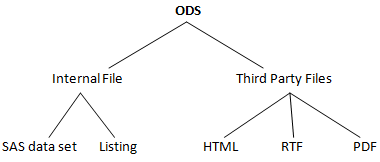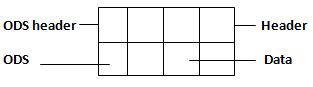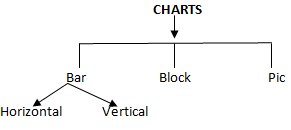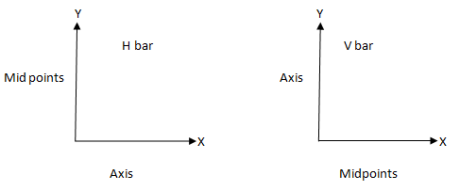# Output Delivery System (ODS) In SAS

Blog Author

Tekslate

Published Date

21st September, 2018

Ratings

Views

140

## Output Delivery System (ODS)

- Using ODS, we can generate reports in required formats. SAS reports we can generate in tables, listings and figures.

- Using ODS concept, we can generate reports in internal files and third party files.### Third Party Files:

1) HTML Reports:

ods listing close;

ods html file='d:\trt.html';

proc print data = trtment;

run;

ods html close;

ods listing;

Ods Options:Style Option:

It can be used to activate required ODS option.

proc sort data = trtment;

by gid;

run;

Eg:   ods listing close;

ods html file='d:\trt.html';

proc print data = trtment;

style(obs) = {foreground = white background = blue}

style(obsheader) = {foreground = white background = red}

style(header) = {foreground = white background = green}

style(data) = {foreground = green background = orange font_weight = bold}}

style(total) = {foreground = blue background = green}

style(grand total) = {foreground = orange background = blue}

sum sub;

by gid;

pageby gid;

title 'This data blongs to #byval(gid);

run;

ods html close;

ods listing;

#(9ash)byval function:

It can be used to supply the values for titles. This function requires only one argument (variable). This argument must be grouping variable.

Byline Option:

It is default global option. It generates bylines in report whenever the report based on by variable or grouping variable.

Var Statement:

It can be used to generate required variables in required colors.

Format Colors:

It can be used to report required data values in required colors.

```At TekSlate, we offer resources to help you learn various IT courses. We avail both written material and demo video
tutorials. For in-depth knowledge and practical experience explore SAS Training.```

/* To report required variables and required data values in required colors */

Eg:          proc format;

value & fcol 'col5mg'='red'

value & fcol 'col6mg'='green'

value & fcol 'col7mg'='black';

value & bcol 'col5mg'='black'

value & bcol 'col6mg'='yellow'

value & bcol 'col7mg'='white';

run;

ods listing close;

ods html file='d:\trt.html';

proc print data = trtment;

style(obs) = {foreground = white background = blue}

style(obsheader) = {foreground = white background = sankar}

style(data) = {font_weight = bold};

var Gid/style = {foreground = gold background = silver};

var drug/style = {foreground = \$fcol background = \$bcol};

var sub/style = {foreground = pink background = green};

run;

ods html close;

ods listing;

### To generate report procedure output in html with ODS concept:

Nowi9ndow Option: It can be used to generate report output window.

2 ODS options for report procedure:

Column: For data

ods listing close;

ods html file='d:\report.html';

proc print data = trtment headline nowd

style(header) = {foreground = red background = green}

style(column) = {foreground = white background = blue font_weight = bold};

column Gid drug sub;

define gid/group;

define drug/group;

define sub/um;

break after gid/ol ul summarize;

rbreak after /dol dul summarize;

compute after gid;

gid = 'Total';

end comp;

run;

ods html close;

ods listing;

/* To report required colors for required variables and data values */

proc format;

value & fcl = 'white'

others = 'brown';

value & bcl = 'black'

others = 'grey';

run;

ods listing close;

ods html file='d:\report.html';

proc print data = trtment headline nowd

style(column) = {font_weight = bold};

column Gid drug sub;

define gid/group style = {foreground=red background=green};

define drug/group style = {foreground=green background=pink};

define sub/sum style = {foreground=&fcl background=&bcl};

break after gid/ol ul summarize style={foreground=white background= blue};

rbreak after /dol dul summarize style={foreground=green background= yellow};

compute after gid;

gid = 'Total';

end comp;

compute after;

gid = 'Gtotal';

end comp;

run;

ods html close;

ods listing;

(Assignment) /* To report required colors for required variables and data values */

proc import datafile = 'd:\comp.xls'

out = comp1 dbms = excel replace

run;

proc format;

value &fcol 'invest' = 'red'

value &fcol 'est_profit' = 'yellow'

value &fcol 'act_profit' = 'pink';

value &bcol 'invest' = 'blue'

value &bcol 'est_profit' = 'green'

value &bcol 'act_profit' = 'black';

run;

ods listing close;

ods html file = 'd:\report.html';

style(column)={foreground=&fcol  background=&bcol};

columns company area (year, _numeric_)

define company/group style={foreground=green  background=pink};

define area/group style={foreground=orange  background=green};

define year/across style={foreground=red  background=yellow};

break after company/ol ul summarize style={foreground=orange background=grey};

rbreak after /dol dul summarize style={foreground=green background=pink};

end comp;

compute est_pro_tot;

est_pro_tot=sum(_C4_ , _C7_);

end comp;

compute act_pro_tot;

act_pro_tot=sum(_C5_ , _C8_);

end comp;

end comp;

compute after company;

company='total&';

end comp;

compute before_page_;

line '   ';

line '   ';

line 'ANALYSIS BELONGS TO SOFTWARE COMPANIES';

line '   ';

end comp;

compute after _page_;

line '   ';

line '   ';

line 'THE INVEST OF ALL COMPANIES';

line '   ';

end comp;

run;

## SAS Graph

Using this module concept we can generate charts and plots.Bar Charts: 2 types

1. Horizontal
2. Vertical

Proc Chart Statement:

Using this statement, we can generate charts. This procedure working based on frequently analysis.

h bar:

Using this, we can generate horizontal bar charts with frequency analysis.

proc freq data=trtment;

table gid;

run;

proc chart data=trtment;              {(or) proc chart data=trtment;

hbar gid/nostats;                             hbar gid/nostats;

run;                                                        vbar gid;

proc chart data=trtment;              }run;

vbar gid;

run;

### nostats Option:

It removes statistical analysis from the report.

### Vbar Statement:

Using this statement, we can generate vertical charts.

Note: We can write multiple hbar & statements and vbar statement in one chart procedure block.

### Options for hbar and Vbar statements

type Option: Using this option, we can indicate required analysis for reporting.

values for type option frequency , cumulative frequency, sum, percent, cumulative percent.

Sum: Using this option, we can indicate analysis variable for sums.

Axis: Using this, we can give scale in graph if it is horizontal graph we get the scale in x-axis if it is vertical graph we will get in y-axis.

/* Total no of patients received drug doses in each group include all visit */

options ls=240;

proc chart data=trtment;

hbar gid/nostats type=sum sumvar=sub

axis=100 to 350 by 5;

run;

### Subgroup option

Using this option, we can indicate subgroup variable for analysis.

In chart graph, SAS indicate to subgroup analysis with graph with symbols (letters).

/* Total no of patients received each drug in each group */

Eg:          proc chart data=trtment;

hbar gid/nostats type=sum sumvar=sub subgroup=drug;

run;

### Midpoints Options

It can be used to give the scale in required axis based on hbar and vbar statements./* Total no of patients received each drug in each visit */

proc chart data=trtment;

hbar week/nostats type=sum

sumvar=sub subgroup=drug

midpoints=0 to 12 by 1;

run;

### Block Charts

Block Statement: It can used to generate block charts

Eg:          proc chart data=treatment;

block drug;

run;

/* Total no of patients taken each drug */

Eg:          proc chart data=treatment;

block drug/type=sum

sumvar=sub;

run;

/* Total no of patients taken each drug in each group */

Eg:          proc chart data=treatment;

block Gid/type=sum

sumvar=sub group=drug;

run;

/* Total no of patients received each drug in each group in each visit */

Eg:          proc chart data=treatment;

hbar Gid/type=sum

sumvar=sub group=drug;

subgroup=week . nostats;

run;

(or)

Eg:          proc chart data=treatment;

block Gid/type=sum

sumvar=sub group=drug;

subgroup=week;

run;

### Pie charts

It can be used to generate pie charts. We can't use group and subgroup options in pie statements.

/* Total no of patients received drug doses in each group */

Eg:          proc chart data=treatment;

pie Gid/type=sum

sumvar=sub;

run;

/* Total no of patients received each drug in each group */

Eg:          proc chart data=treatment;

pie Gid/type=sum

sumvar=sub;

by drug;

run;

- In pie charts, dependent analysis we can be do by using by statement instead of group and subgroup option.

### gchart procedure:

It can be used to generate the charts in graph window(horizontal, vertical, pie -we can generate but block charts we can't run with gchart procedure.)

Eg:          proc gchart data=treatment;

pie Gid/type=sum

sumvar=sub;

run;

Eg:          proc gchart data=treatment;

pie Gid/type=sum

sumvar=sub;

group=drug subgroup=week;

run;

Plots:

Plot Statement: It can be used to generate plots.

Syntax: Plot yaxis variable * xaxis variable 7 reporting variable;

Eg:          proc plot data=treatment;

plot gid * drug;

run;

/* No of patients receivedeach drug in each group */

proc plot data=treatment;

plot gid * drug & sub;

run;

For indepth knowledge on SAS, click on below